Thermal Strain

# Linear coefficient of thermal expansion:

An unloaded uniform bar will extend due to a uniform change in the temperature. This extension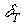is linearly proportional to the temperature change and the strain associated with it is given by the relation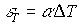where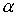is the linear coefficient of thermal expansion and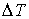is the rise in temperature.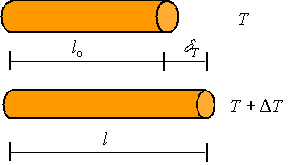The strain is given by the extension divided by the original length through the standard relation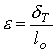Combining this and the above expression one gets the relation for the extension of a uniform bar under a uniform change in temperature as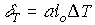For a non-uniform bar or a non-uniform temperature distribution in the bar, one needs to integrate the strain using the expression for extension of a non-uniform bar to get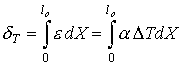# Volumetric coefficient of thermal expansion:

The volumetric strain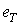is linearly proportional to the change in temperature and is given by the relation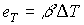where the coefficient of proportionality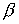is the volumetric coefficient of thermal expansion.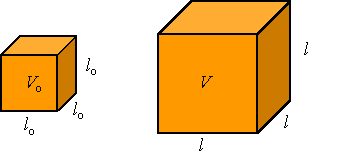The volumetric coefficient of thermal expansion  is related to the linear  coefficient of thermal expansion through the relation between the volumetric strain and the axial strain. The volumetric strain e is given as the change in volume divided by the original volume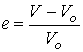For a cube of isotropic material (a material which behaves the same along all different directions) with initial sides of length lo, the initial volume is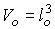and since each side extends an amount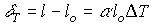, the new volume is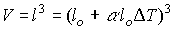. Substituting these expressions into the above relation one gets for small thermal strains the approximate relation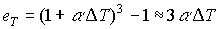Therefore, for small thermal strains in an isotropic material the relation between the linear coefficient of thermal expansion and the volumetric coefficient of thermal expansion is given by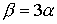# Combining thermal and mechanical loads:

Since we only are considering linear theories, it is assumed that the thermal and mechanical effects are additive in the sense that the total strain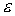in a bar is the addition of the thermal strain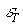and the mechanical strain. For a uniform bar under both uniform thermal and mechanical loading, this can be written as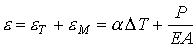which results in the total extension being given by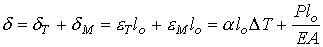In the case of non-uniform members, one simply integrated the expression for strain once for the thermal strain and then again for the mechanical strain to get, respectively, the expression for the extension due to thermal expansion and the expression for the extension due to mechanical loading. Again, the sum of these expressions is the total extension of the bar